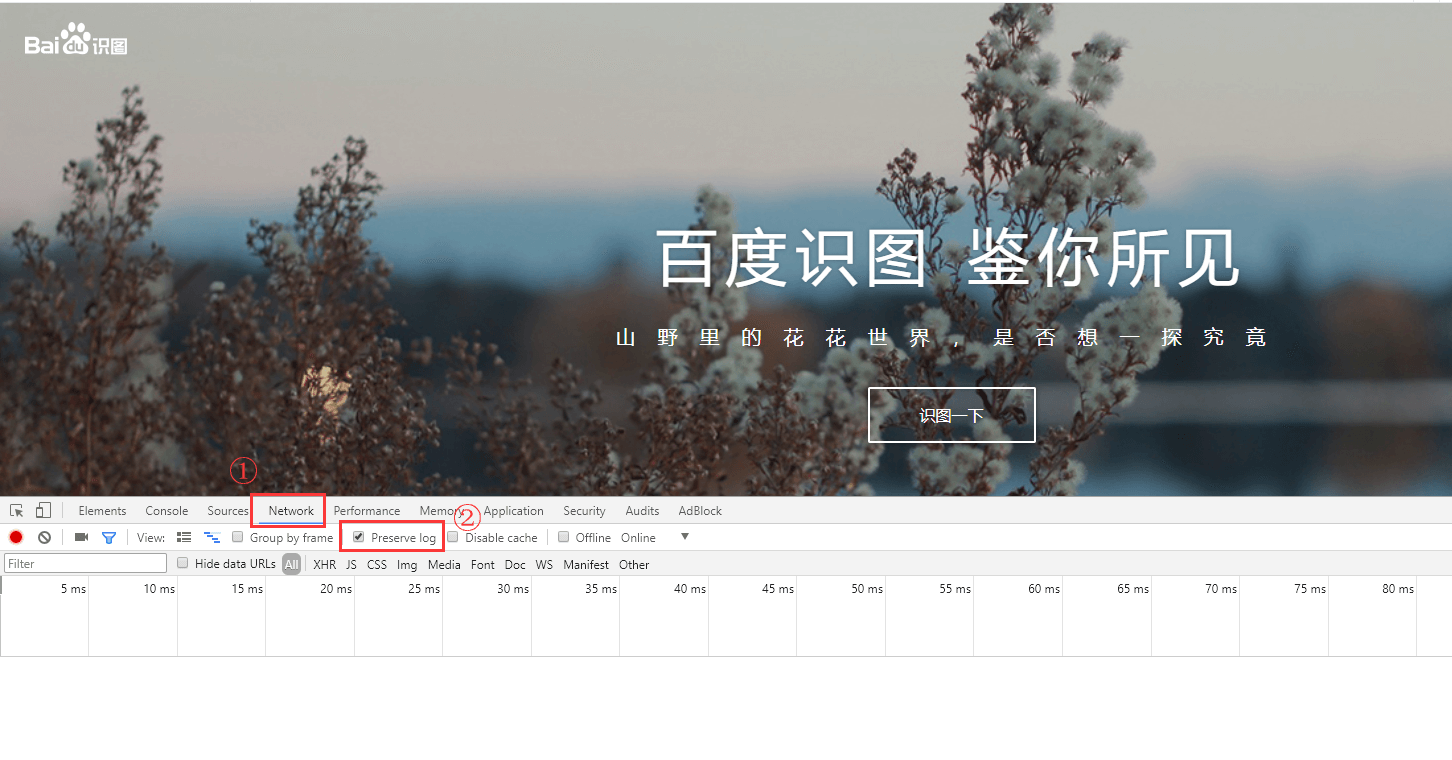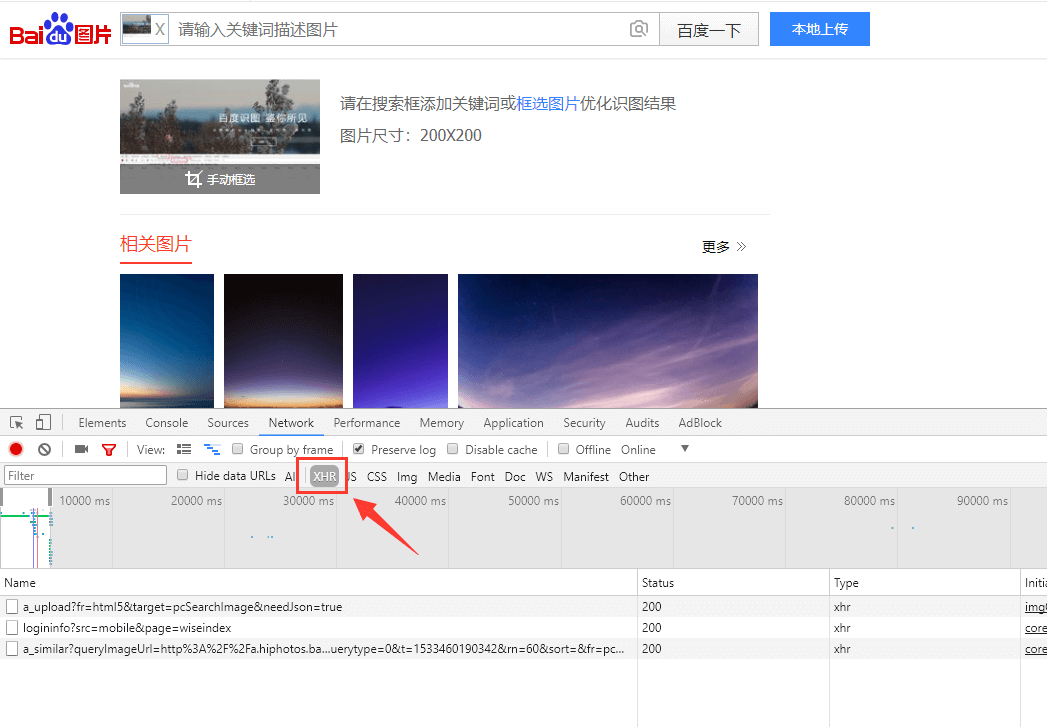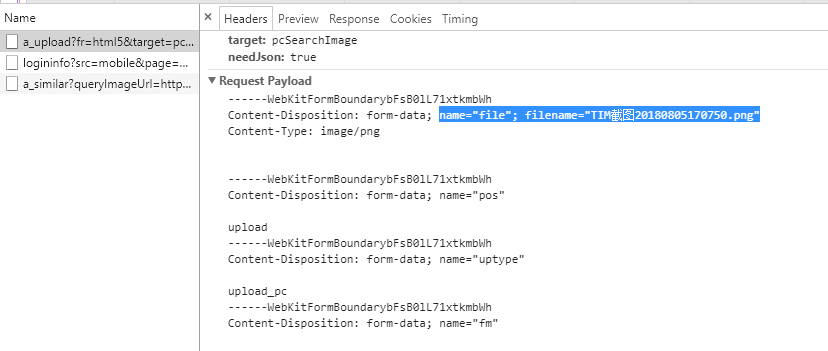# PHP利用百度当图床

mengkun 22.6K*上面这张图片是我两年前上传至百度识图的，至今依然有效

## 抓接口http://image.baidu.com/pcdutu/a_upload?fr=html5&target=pcSearchImage&needJson=true

## 写代码

<?php

/**
* 上传图片到百度识图接口，获取图片外链
*
* @param     $file 图片文件 * @return 图片链接(上传成功) NULL(上传失败) * @copyright (c) mengkun(https://mkblog.cn/1619/) */ function uploadToBaidu($file) {
// API 接口地址
$url = 'http://image.baidu.com/pcdutu/a_upload?fr=html5&target=pcSearchImage&needJson=true'; // 文件不存在 if(!file_exists($file)) return '';

// POST 文件
if (class_exists('CURLFile')) {     // php 5.5
$post['file'] = new CURLFile(realpath($file));
} else {
$post['file'] = '@'.realpath($file);
}

// CURL 模拟提交
$ch = curl_init(); curl_setopt($ch, CURLOPT_URL , $url); curl_setopt($ch, CURLOPT_RETURNTRANSFER, 1);
curl_setopt($ch, CURLOPT_POST, 1); curl_setopt($ch, CURLOPT_POSTFIELDS, $post);$output = curl_exec($ch); curl_close($ch);

// 返回结果为空（上传失败）
if($output == '') return ''; // 解析数据$output = json_decode($output, true); if(isset($output['url']) && $output['url'] != '') { return$output['url'];
}
return '';
}

// 使用示例：
$url = uploadToBaidu('1.jpg'); echo$url;

## 课后练习

1.X光影 Lv 1

优秀

2.尹深 Lv 1

这个失效了吧

3.迟早要还的____________________ Lv 1

大神，如何才能从完成新手入门，太难了